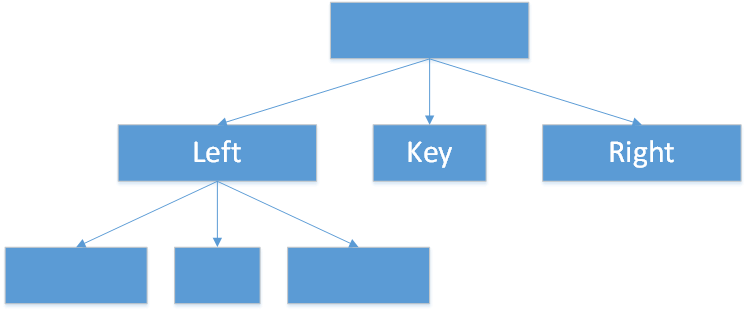```<?php
//递归函数的特点有两个
//1.要有递归出口,应该放到递归函数的最前面
//2.要有递归调用
function quick sort (\$arr) {
//递归出口判断要放在最前面
if (count(\$arr) <= 1){
return \$arr;
}
//把第一个元素从数组中弹出去放在一个变量中
\$key = array_shift(\$arr);
//把刚才弹出的第一个元素(基准元素)存到一个数组中
\$key_arr = array(\$key);
//定义两个空数组，给左边和右边的数组进行初始化。
\$left_arr = array();
\$right_arr = array();
foreach (\$arr as \$value){
if(\$value < \$key){
\$left_arr[] = \$value;
}else{
\$right_arr[] = \$value;
}
}
//这里就是递归调用
return array_merge(quick_sort(\$left_arr), \$key_arr, quick_sort(\$right_arr));
}```